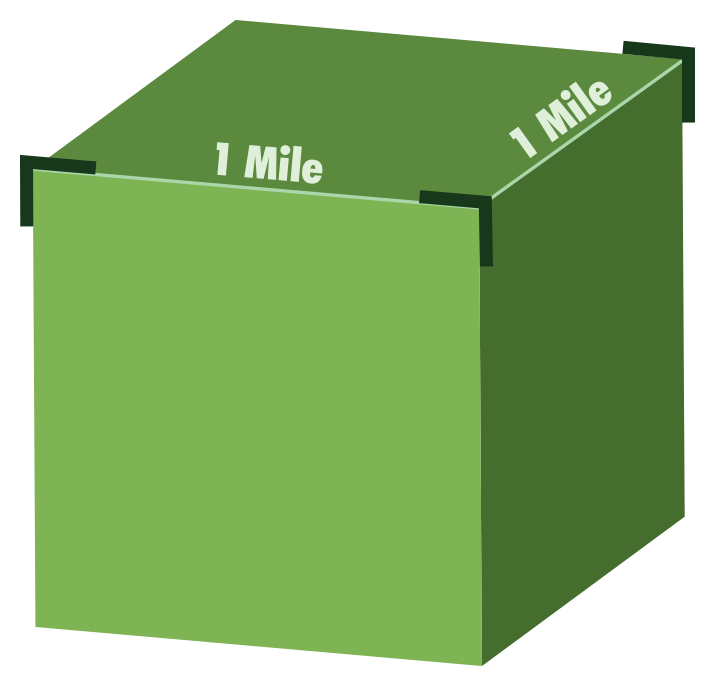# Where Do We Find Fossil Fuels?

## Where petroleum and natural gas are found:Cubic Mile
• A square mile is a square of land that measures one mile on each side. One square mile equals 640 acres. So how can we picture the size of the Bakken?  Since it occupies about 145,000 square miles, we can multiply that number by 640 to get the number of acres it occupies (145,000 x 640 = 92,800,000). The length and width of the Bakken is about the same size as 93 million football fields.
• The part of the Bakken in western North Dakota that currently produces oil underlies about 12,000 square miles (12,000 x 640 = 7,680,000 acres). The North Dakota Bakken is almost the size of 8 million football fields.